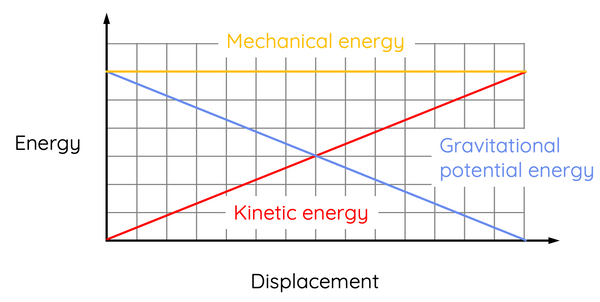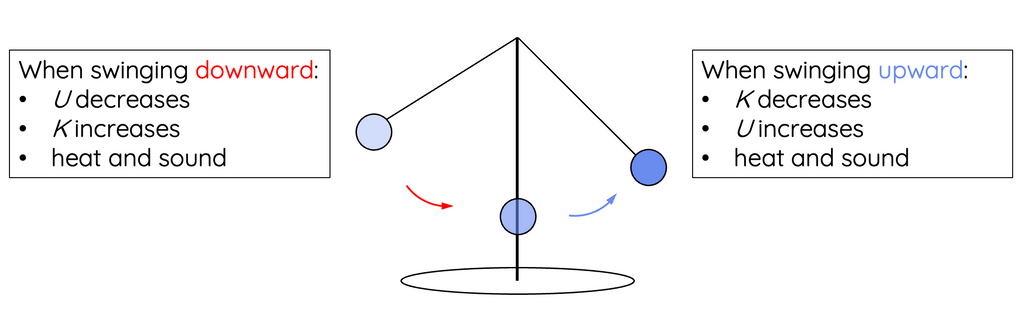# Law of Conservation of Energy

This topic is part of the HSC Physics course under the section Forces, Acceleration and Energy.

### HSC Physics Syllabus

• apply the special case of conservation of mechanical energy to the quantitative analysis of motion involving:

– work done and change in the kinetic energy of an object undergoing accelerated rectilinear motion in one dimension 𝑊=𝐹𝑠=𝐹𝑠cos𝜃
– changes in gravitational potential energy of an object in a uniform field ∆U = mg∆h

### What is Conservation of Energy?

This explores the law of conservation of energy as well as the conservation of mechanical energy.

### Law of Conservation of Energy

One of the fundamental principles of physics is the Law of Conservation of Energy, which states that energy cannot be created or destroyed but only transferred or transformed.

The Law of Conservation of Energy asserts that the total energy of an isolated system remains constant, no matter what processes are occurring within it. This law is crucial in physics because it allows us to create equations and make predictions about the behaviour of physical systems.

### Conservation of Mechanical Energy

Conservation of Mechanical Energy is a principle that applies to a specific type of system in physics. The mechanical energy of a system is the sum of its kinetic energy and potential energy.

The Law of Conservation of Mechanical Energy states that the total mechanical energy in a closed system—meaning one without any external forces doing work on the system, such as friction or air resistance—is conserved. In other words, the total mechanical energy remains constant over time.This means that when an object's kinetic energy increases, its potential energy decreases by an equivalent amount, and vice versa. This is given by the following equations.

$$\Delta U = -\Delta K$$

$$mg\Delta h = -(\frac{1}{2}mv^2 - \frac{1}{2}mu^2)$$

$$g\Delta h = \frac{1}{2} (u^2 - v^2)$$This can occur, for example, when a mass is falling under the influence of gravity in the absence of air resistance. As the mass falls, its vertical displacement from the ground decreases. As a result, its gravitational potential energy decreases, and kinetic energy increases (by the same amount). Since its kinetic energy increases, its speed increases.

### Conservation of Mechanical Energy – Calculation Example

A ball is dropped from rest at a height 5 m above the ground. Calculate the change in gravitational potential energy and the speed of the ball when it strikes the ground.

$$\Delta U = -\Delta K$$

$$mg\Delta h = -(\frac{1}{2}mv^2 - \frac{1}{2}mu^2)$$

$$g\Delta h = \frac{1}{2} (u^2 - v^2)$$

$$(9.8)(0-5) = \frac{1}{2} (0^2 - v^2)$$

$$v = 9.9 \hspace{2mm} m \hspace{1mm} s^{-1}$$

Since the mass is not in the above equation, the final velocity of the ball is independent of its mass. If the ball is dropped from a height greater than 5 m, then its final kinetic energy and velocity will also be greater.

### When is Mechanical Energy Not Conserved?

It's important to note that mechanical energy conservation only holds in systems where all the forces doing work are conservative forces, like gravity or the elastic force in a spring. If non-conservative forces like friction or air resistance are significant, they will convert some mechanical energy into heat or other forms of energy, so the total mechanical energy will not be conserved.

For example, consider a swinging pendulum:As the pendulum swings downward, its gravitational potential energy is transformed into kinetic energy as well as heat and sound due to the presence of friction and air resistance.

As the pendulum swings upward, its kinetic energy is transformed back into gravitational potential energy. However, the amount gravitational potential energy of the pendulum will never return to its original value due to partial transformation into heat and sound. This explains why the height of the pendulum decreases throughout its motion, eventually coming to rest.

Although the pendulum's mechanical energy is not conserved, the total energy of the system (kinetic energy, potential energy, heat and sound) is still conserved regardless of the presence of non-conservative forces.